# NEET Physics Video Lectures

Now that you have decided to take a deep dive into the world of medicine, the next propelling thing which you can be offered are the best physics video lectures for NEET. Over the years it has been observed that the NEET aspirants face a turmoil when it comes to handling physics from examination point of view. This is because many of the aspirants are under the delusion that physics will not be useful in their choice of profession, which is wrong to every extent. And the rest are caught up in the nightmare of having to study the complicated subject of physics, which they detest.

Hence, CLEAREXAM has taken up the baton to do away with all these delusions and nightmares by making physics an enjoyable experience for the NEET aspirants. The Video lectures of Physics have been prepared by top-IITians and their teaching faculty. CLEAREXAM’s team of subject experts have burnt the midnight oil to compile all the NEET Physics video lectures and divide them topic-wise, in order to make it easier for the student’s comprehension.

Now by the latest stats, Motion of system of particles and rigid body, Magnetic effects of current and magnetism, Electromagnetic waves comprise of 5% weightage respectively. Thermodynamics, Electrostatics and Electronic Devices have a weightage of 9% respectively. Optics itself occupies 10% of the marks, whereas Current Electricity, EMI and AC take up 8% of the whole marks of the NEET respectively.

These lectures are accessible on mobile-phones and laptops with an internet connection. The motive of providing the NEET video lectures for physics is to remove all the conceptual doubts of the students and make the physics section much more scoring for them. CLEAREXAM wishes all the students, Best of Luck, and hopes that this endeavour by the team of CLEAREXAM is well received.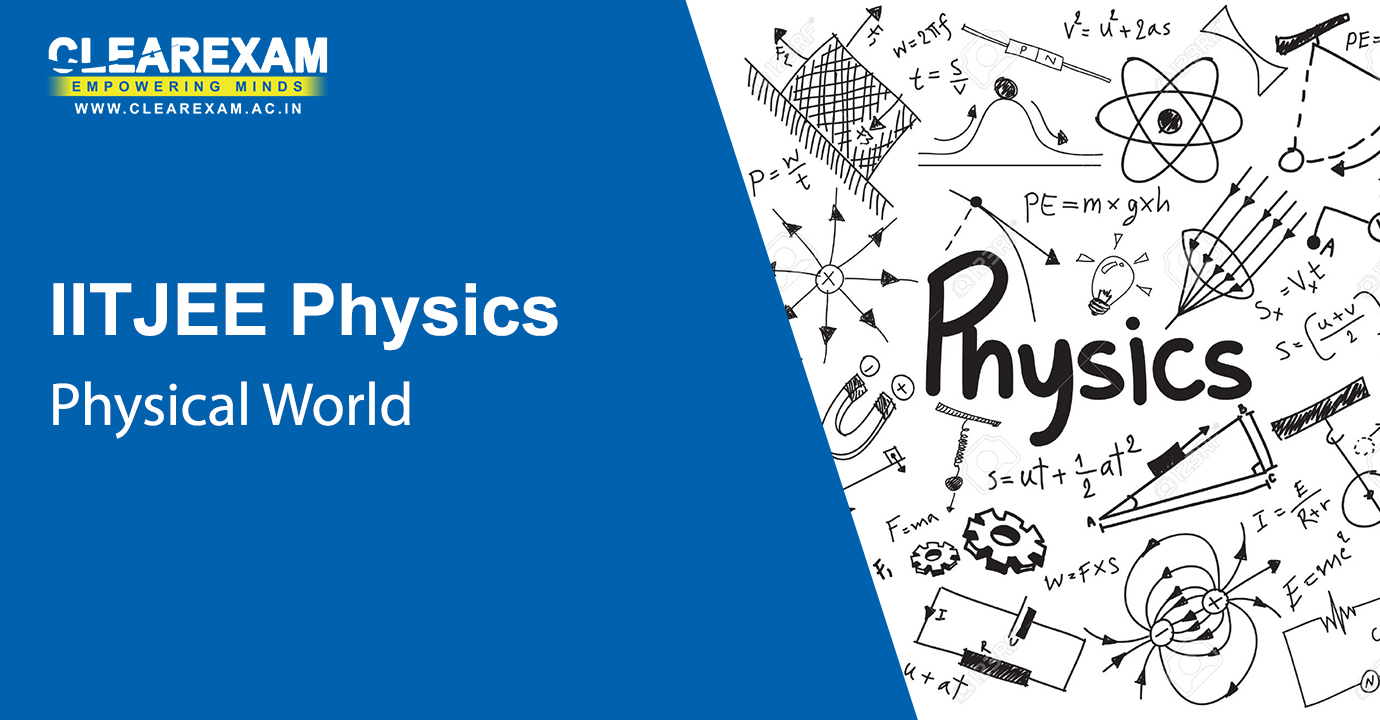Physical World
Make a head start by knowing the physical world around you with the NEET Physics Physical World Video Lectures. The topics comprise of Definition of physics, Excitement of physics, fundamental forces in nature, physics, technology & society and nature of physical laws.
Physical World
Chapter - Physical World
Subject - NEET Physics
Excitement in Physics
Chapter - Physical World
Subject - NEET PhysicsUnits and Measurements
Check out the NEET Physics Units and Measurements Video Lectures on the topics which follow; international system of units, measurement of length, mass & time, precision of instruments & errors in measurement, significant figures, dimensions of physical quantities, dimensional formulae & dimensional equations, dimensional analysis & its applications. These videos of 1 hour each will help you clear your basic concepts.
Dimensions of Physical Quantities, Dimensional Analysis and its Applications
Chapter - Units and Measurements
Subject - NEET Physics
Introduction to Error Analysis
Chapter - Units and Measurements
Subject - NEET Physics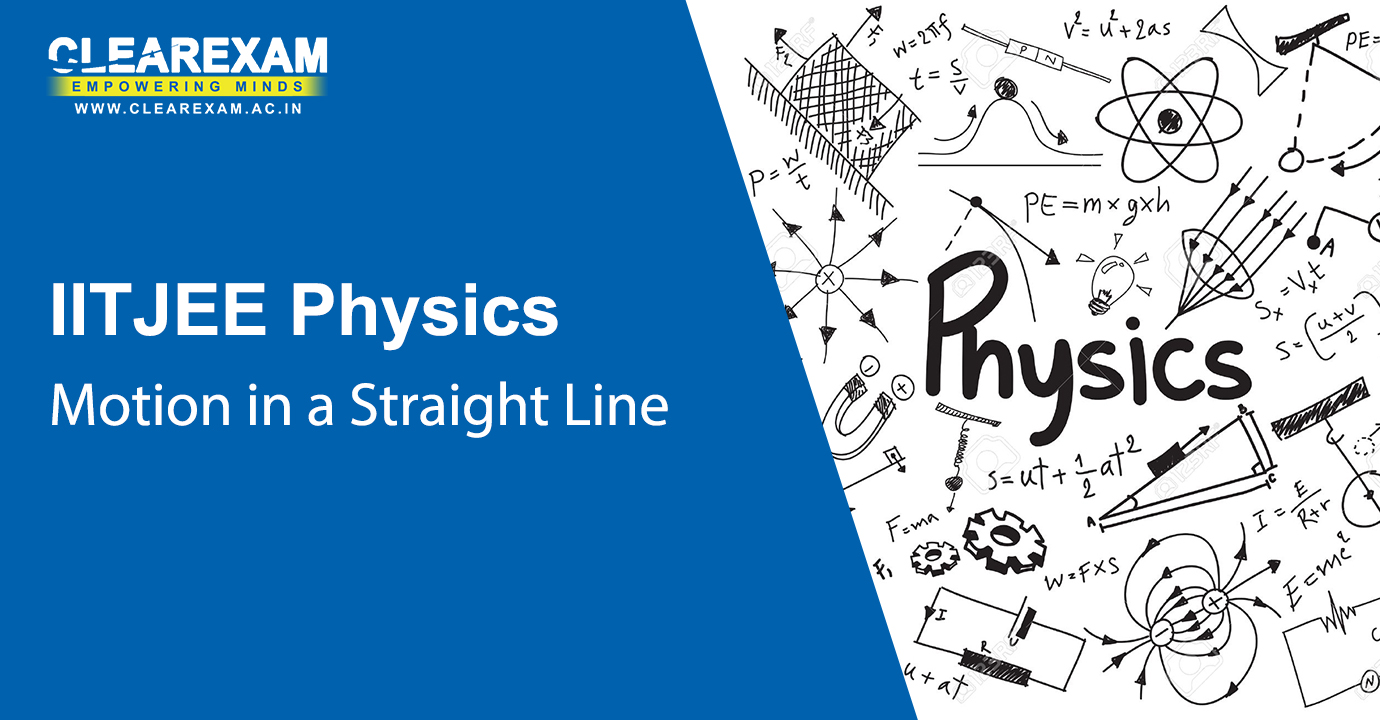Motion in a Straight Line
Learn the Unit: Motion in a straight line, in an interactive manner through NEET Physics Motion in a Straight Line Video Lectures. It covers the topics as given : position, path length & displacement, average velocity & average speed, instantaneous velocity & speed, acceleration, kinematic equations for uniformly accelerated motion and relative velocity.
Introduction to Kinematics: Basic Mathematical Concepts
Chapter - Motion in a Straight Line
Subject - NEET Physics
Relative Velocity and Examples
Chapter - Motion in a Straight Line
Subject - NEET PhysicsMotion in a Plane
Learn the Motion in a Plane in an engaging manner through the NEET Physics Motion in a Plane Video Lectures whichrefers to topics like scalars & vectors, multiplication of vectors by real numbers, addition & subtraction of vectors in graphical method, resolution of vectors, vector addition in analytical method, motion in a plane, motion in a plane with constant acceleration, relative velocity in two dimensions, projectile motion and uniform circular motion.
Introduction to Vectors
Chapter - Motion in a Plane
Subject - NEET Physics
Introduction to Vector Operations
Chapter - Motion in a Plane
Subject - NEET Physics
Planer Motion
Chapter - Motion in a Plane
Subject - NEET Physics
Problems in Kinematics
Chapter - Motion in a Plane
Subject - NEET PhysicsLaws of Motion
Learn Laws of Motion from the masters themselves. In the given NEET Physics Laws of Motion Video Lectures, curated by the best of the best subject experts, the following topics are covered :  Aristotle’s Fallacy, the law of inertia, Newton's first law of motion, Newton’s second law of motion, Newton’s third law of motion, conservation of momentum, equilibrium of a particle, common forces in mechanics, circular motion and solving problems in mechanics.
Newton's First Law of Motion
Chapter - Laws of Motion
Subject - NEET Physics
Newton's Second and Third Laws of Motion
Chapter - Laws of Motion
Subject - NEET Physics
Problem Solving Newton's Second Law of Motion
Chapter - Laws of Motion
Subject - NEET Physics
Forces on Bodies: Contact and Non-Contact Forces
Chapter - Laws of Motion
Subject - NEET Physics
Forces on Bodies: Systems involving Strings or Springs
Chapter - Laws of Motion
Subject - NEET Physics
Forces on Bodies: Procedure to Solve Problems
Chapter - Laws of Motion
Subject - NEET Physics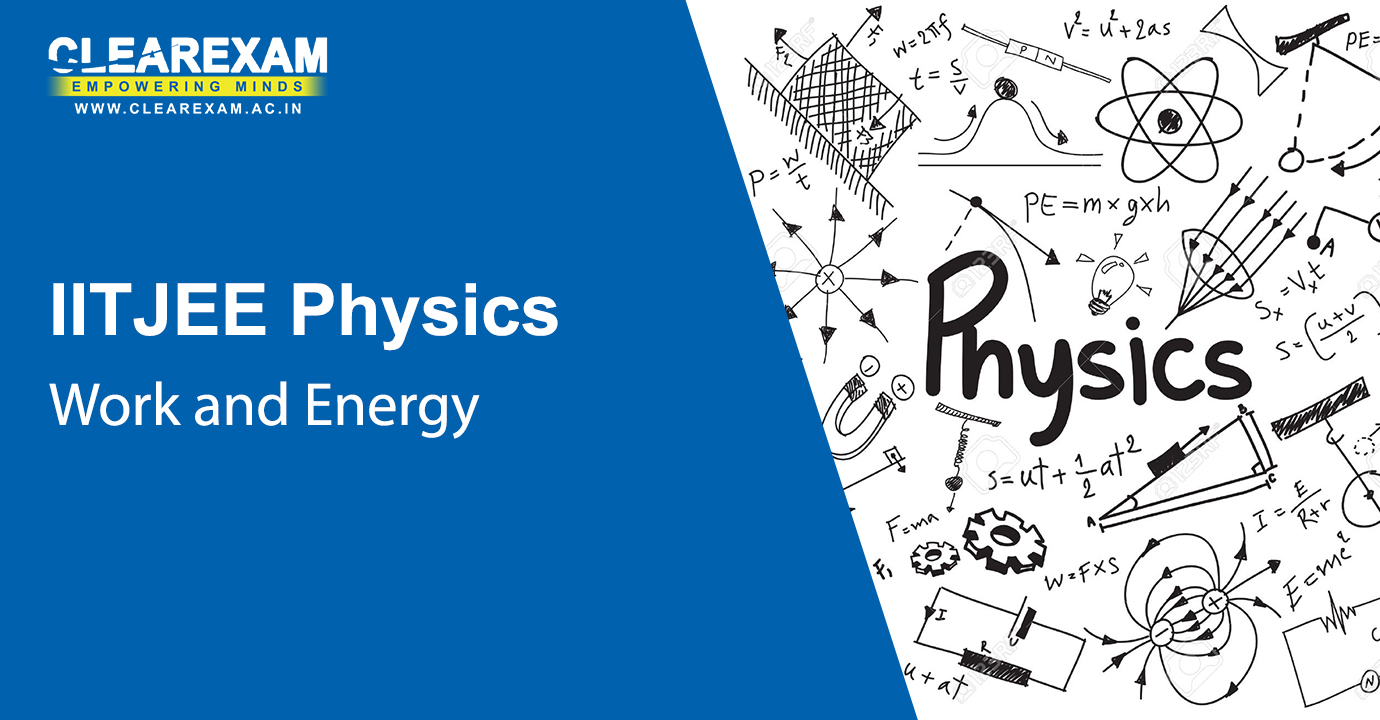Work and Energy
Let your energy throttle with these NEET Physics Work and Energy Video Lectures , covering the following topics; notions of work and kinetic energy, work, kinetic energy, work done by a variable force, work-energy theorem for a variable force, concept of potential energy, conservation of mechanical energy, potential energy of a spring, the law of conservation of energy, power and collisions.
Constant and Variable Forces, Kinetic Energy
Chapter - Work and Energy
Subject - NEET Physics
Work energy and Impulse Momentum Principles, Conservation of Momentum
Chapter - Work and Energy
Subject - NEET Physics
Impact and Collision
Chapter - Work and Energy
Subject - NEET Physics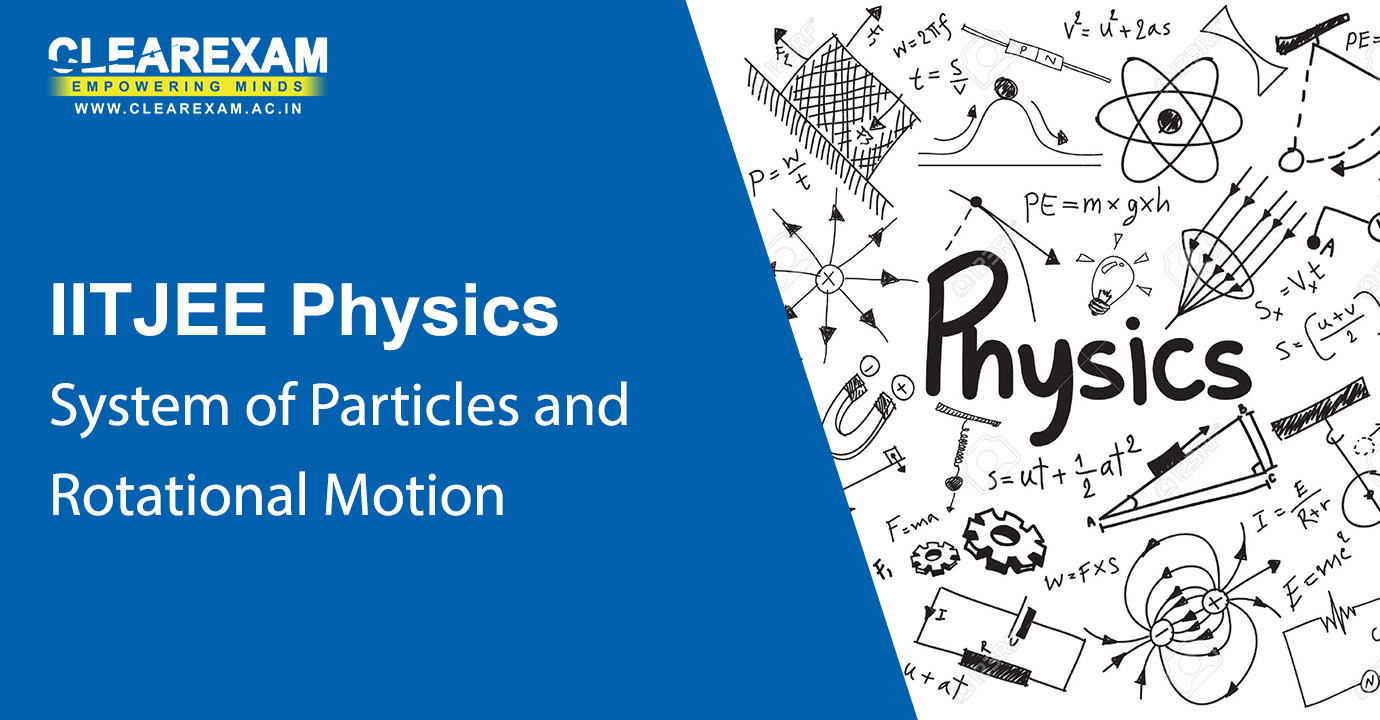System of Particles and Rotational Motion
Learn the unit System of particles and rotational motion in a fun way. The NEET Physics System of Particles and Rotational Motion Video Lecturescomprises of topics such as centre of mass, motion of centre of mass, linear momentum of a system of particles, vector product of two vectors, angular velocity & its relation with linear velocity, torque & angular momentum, equilibrium of a rigid body, moment of inertia, theorems of perpendicular & parallel axes, kinematics of rotational motion about a fixed axis, dynamics of rotational motion about a fixed axis, angular momentum in case of rotation about a fixed axis and rolling motion.
Introduction, Center of Mass
Chapter - System of Particles and Rotational Motion
Subject - NEET Physics
Motion of Center of Mass, Relative Motion and Reduced Mass
Chapter - System of Particles and Rotational Motion
Subject - NEET Physics
Vector Products, Angular Velocity and Angular Acceleration
Chapter - System of Particles and Rotational Motion
Subject - NEET Physics
Torque and Angular Momentum
Chapter - System of Particles and Rotational Motion
Subject - NEET Physics
Equilibrium of a Rigid Body, Moments and Center of Gravity
Chapter - System of Particles and Rotational Motion
Subject - NEET Physics
Moment of Inertia, Theorems of Perpendicular and Parallel Axes
Chapter - System of Particles and Rotational Motion
Subject - NEET Physics
Rotational motion about a Fixed Axis - Kinematics and Dynamics
Chapter - System of Particles and Rotational Motion
Subject - NEET Physics
Rotational motion about a Fixed Axis - Angular Momentum
Chapter - System of Particles and Rotational Motion
Subject - NEET Physics
Problem Session-1: Motion of System of Particles and Rigid Bodies
Chapter - System of Particles and Rotational Motion
Subject - NEET Physics
Problem Session-2: Motion of System of Particles and Rigid Bodies
Chapter - System of Particles and Rotational Motion
Subject - NEET PhysicsGravitation
Relive gravitation with the best minds. The NEET Physics Gravitation Video Lectures on kepler’s laws, universal law of gravitation, the gravitational constant, acceleration due to gravity of the earth, acceleration due to gravity below & above the surface of earth, gravitational potential energy, escape speed, earth satellites, energy of an orbiting satellite, geostationary & polar satellites and weightlessness, are very comprehensible.
Introduction to Gravitation
Chapter - Gravitation
Subject - NEET Physics
Conservation Laws, Fundamental Forces, Estimation of Distances
Chapter - Gravitation
Subject - NEET Physics
More on Distances, Galilean Law, Kepler Laws, Centripetal Forces
Chapter - Gravitation
Subject - NEET Physics
Galilean Law, Kepler Laws, Centripetal Forces, Gravitational Law
Chapter - Gravitation
Subject - NEET Physics
Determination of Gravitational Constant (G)
Chapter - Gravitation
Subject - NEET Physics
Tidal Forces, Gravitational Potential Energy, Energy Conservation
Chapter - Gravitation
Subject - NEET Physics
Gravitational Potential and Potential Energy
Chapter - Gravitation
Subject - NEET Physics
Escape Velocity, Natural and Artificial Satellites
Chapter - Gravitation
Subject - NEET Physics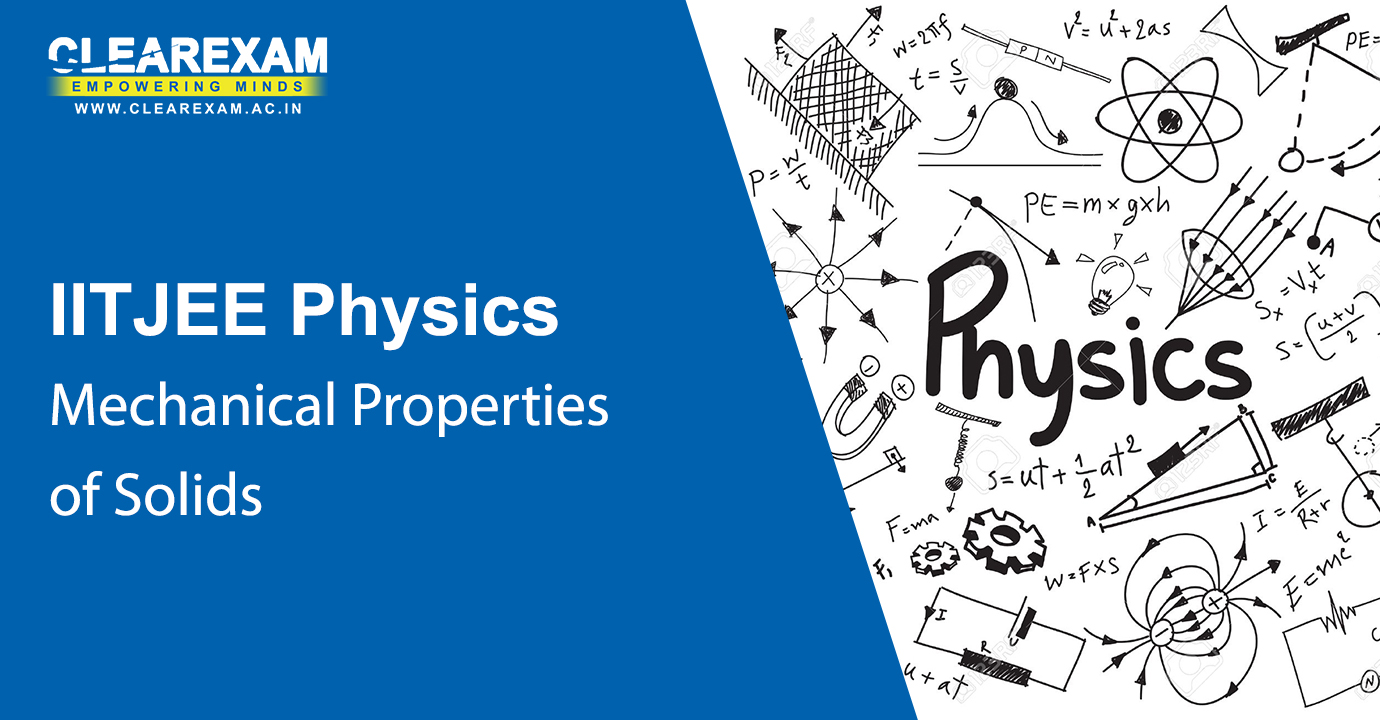Mechanical Properties of Solids
These 1 hour  NEET Physics Mechanical Properties of Solids Video Lectures consisting of the topics such as elastic behaviour of solids, stress & strain, Hooke’s law, stress-strain curve, elastic moduli and applications of elastic behaviour of materials are very informative. Do take a look.
Elastic Behaviour of Solids, Modulus of Elasticity, Stress-Strain, Hooke's Law
Chapter - Mechanical Properties of Solids
Subject - NEET Physics
Experimental Determination of Young's Modulus
Chapter - Mechanical Properties of Solids
Subject - NEET Physics
Elastic Properties of Human Body
Chapter - Mechanical Properties of Solids
Subject - NEET PhysicsMechanical Properties of Fluids
Get your basics of the Mechanical properties of Fluids cleared. Go through the NEET Physics Mechanical Properties of Fluids Video Lectures having the topics such as Pressure, Streamline Flow, Bernoulli’s Principle, Viscosity, Reynolds Number and Surface Tension.
Density, Pressure due to Static Fluids
Chapter - Mechanical Properties of Fluids
Subject - NEET Physics
Atmospheric Pressure and Gauge Pressure, Measurement of Pressure
Chapter - Mechanical Properties of Fluids
Subject - NEET Physics
Pascal's Law, Buoyancy and Archimedes Principle, Streamline Flow, Equation of Continuity
Chapter - Mechanical Properties of Fluids
Subject - NEET Physics
Bernoulli's Principle, Venturimeter, Surface Tension
Chapter - Mechanical Properties of Fluids
Subject - NEET Physics
Viscosity, Poiseuille's Law
Chapter - Mechanical Properties of Fluids
Subject - NEET PhysicsKinetic Theory of Gases
These 1 hour video lectures titled NEET Physics Kinetic Theory of Gases Video Lectures have topics such as molecular nature of matter, behaviour of gases, kinetic theory of an ideal gas, law of equipartition of energy, specific heat capacity and mean free path explained in a detailed manner by the best of subject experts.
Microscopic and Macroscopic Apporach to Thermal Properties
Chapter - Kinetic Theory of Gases
Subject - NEET Physics
Kinetic Theory of Gases & Equation of Ideal Gas
Chapter - Kinetic Theory of Gases
Subject - NEET Physics
Equipartition of Energy
Chapter - Kinetic Theory of Gases
Subject - NEET Physics
Mean Freepath and Non-Ideal Gas
Chapter - Kinetic Theory of Gases
Subject - NEET Physics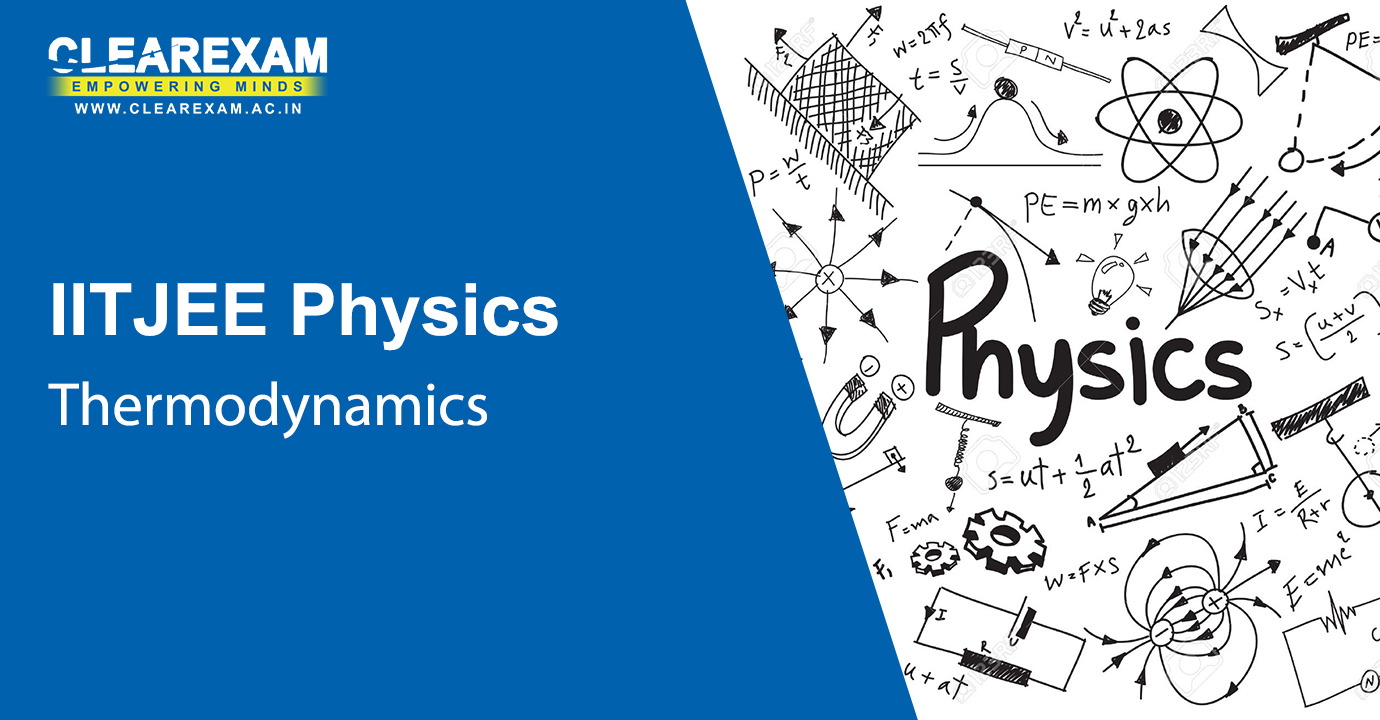Thermodynamics
The topic of Thermodynamics is very relatable by one and all in their daily lives. Under the NEET Physics Thermodynamics Video Lectures, thereare topics like thermal equilibrium, the zeroth law of thermodynamics, heat, internal energy & work, first law of thermodynamics, specific heat capacity, thermodynamic state variables & equation of state, thermodynamic processes, heat engines, refrigerators & heat pumps, second law of thermodynamics, reversible & irreversible processes and carnot engine.
Introduction to Thermodynamics - First Law and Internal Energy
Chapter - Thermodynamics
Subject - NEET Physics
First Law: Work Done in Different Thermodynamics Processes
Chapter - Thermodynamics
Subject - NEET Physics
Heat Engine and Refrigerators
Chapter - Thermodynamics
Subject - NEET Physics
Carnot Engine and Carnot Theorem
Chapter - Thermodynamics
Subject - NEET Physics
Entropy and T-S Diagram
Chapter - Thermodynamics
Subject - NEET Physics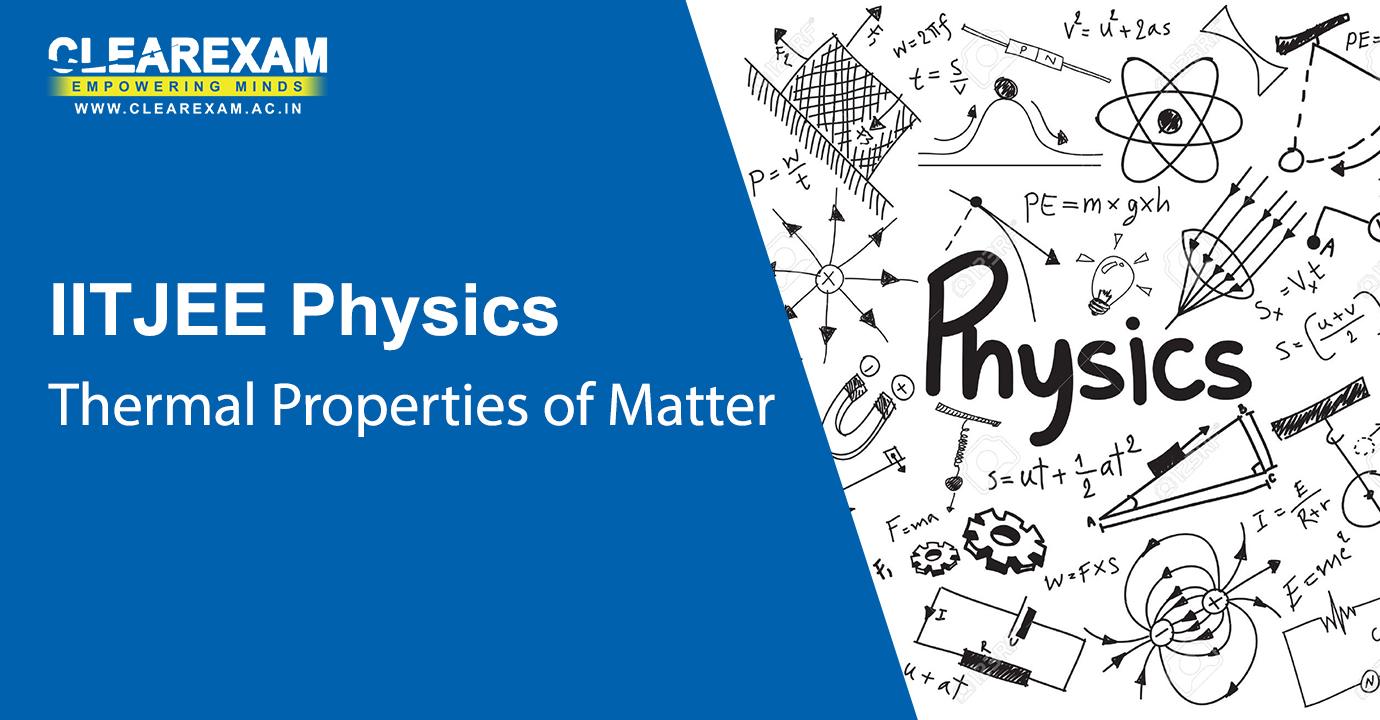Thermal Properties of Matter
Refer to the NEET Physics Thermal Properties of Matter Video Lectures consisting of the topics such as temperature & heat, measurement of temperature, ideal-gas equation & absolute temperature, thermal expansion, specific heat capacity, calorimetry, change of state, heat transfer and Newton’s law of cooling, for a thorough revision.
Concept of Temperature, Thermal Expansion
Chapter - Thermal Properties of Matter
Subject - NEET Physics
Specific Heat Capacity, Calorimetry, Change of State
Chapter - Thermal Properties of Matter
Subject - NEET Physics
Heat Transfer, Newton's Law of Cooling
Chapter - Thermal Properties of Matter
Subject - NEET Physics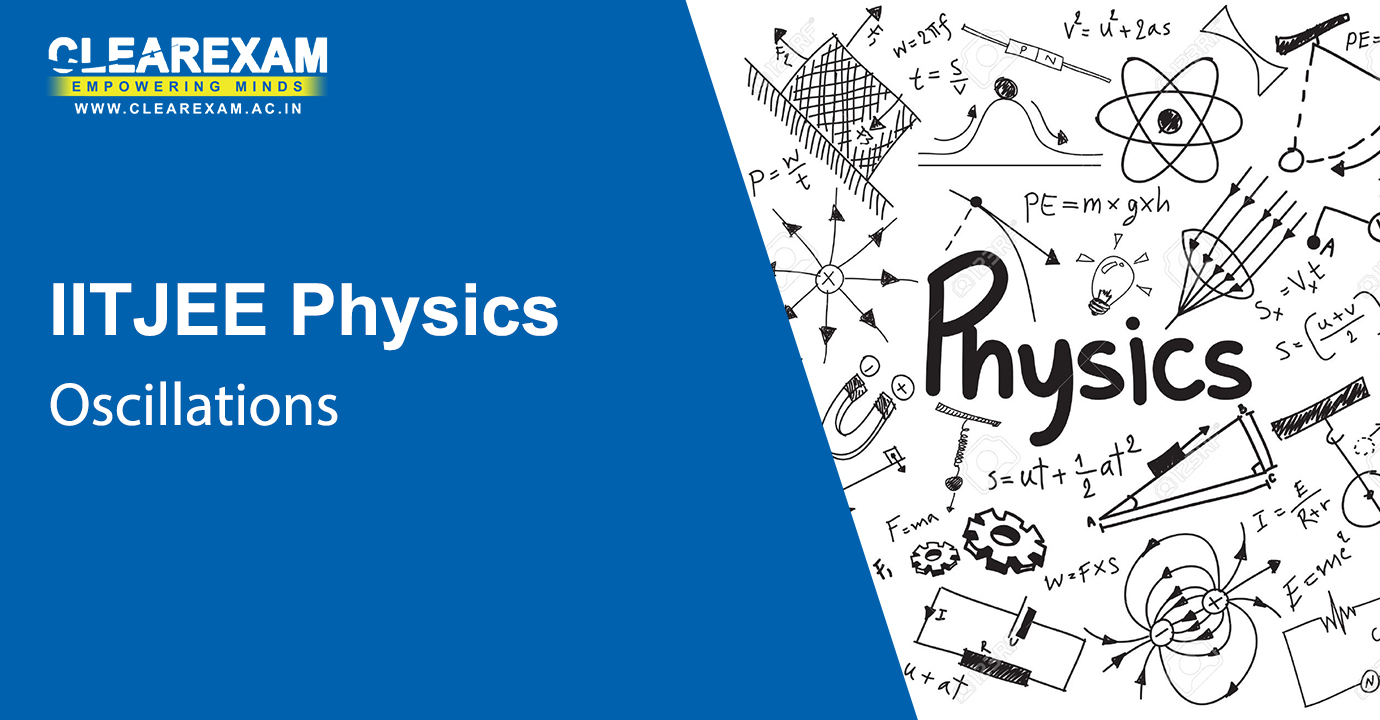Oscillations
Indulge in a periodic revision of the Oscillations topic through the NEET Physics Oscillations Video Lectures which has topics like periodic & oscillatory motions, simple harmonic motion, simple harmonic motion & uniform circular motion, velocity & acceleration in simple harmonic motion, force law for simple harmonic motion, energy in simple harmonic motion, some systems executing SHM, damped simple harmonic motion and forced oscillations & resonance.
Introduction to Periodic Motion
Chapter - Oscillations
Subject - NEET Physics
Introduction to Simple Harmonic Motion
Chapter - Oscillations
Subject - NEET Physics
Examples of Simple Harmonic Motion
Chapter - Oscillations
Subject - NEET Physics
Damped Harmonic Oscillator
Chapter - Oscillations
Subject - NEET PhysicsWaves
Swim through the Waves as the best of the minds help you comprehend this topic through the NEET Physics Waves Video Lectures which talk about the transverse & longitudinal waves, displacement relation in a progressive wave, the speed of a travelling wave, the principle of superposition of waves, reflection of waves, beats and Doppler Effect.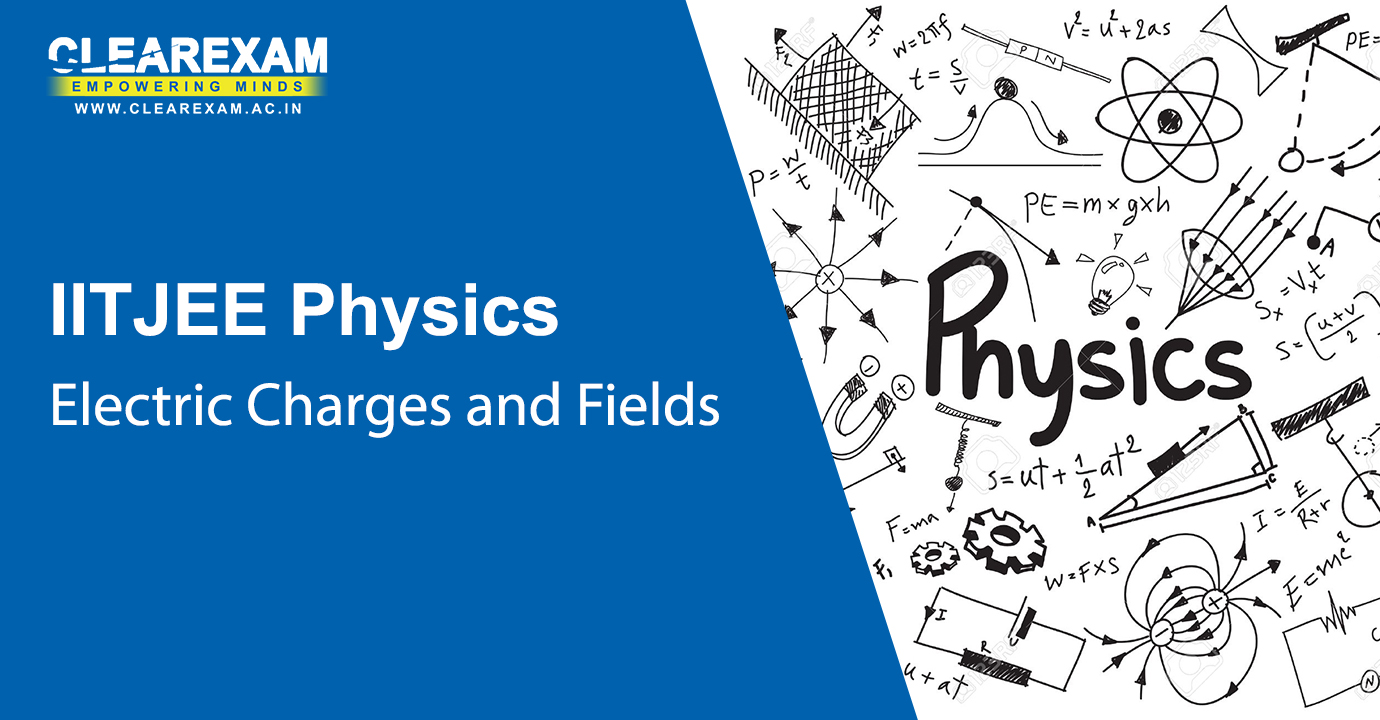Electric Charges and Fields
Comprehension of the topic Electric charges and Fields has never been made easy. NEET Physics Electric Charges and Fields Video Lectures contain the topics such as electric charges, conductors & insulators, charging by induction, basic properties of electric charge, Coulomb's Law, forces between multiple charges, electric field, electric field lines, electric flux, electric dipole, dipole in a uniform external field, continuous charge distribution, Gauss’s Law, and Application of Gauss’s Law.
Concept of Charge and Coulomb's Law
Chapter - Electric Charges and Fields
Subject - NEET Physics
Superposition Principle for Electrostatic Force
Chapter - Electric Charges and Fields
Subject - NEET Physics
Concept of Electric Field, Electric Dipole
Chapter - Electric Charges and Fields
Subject - NEET Physics
Field due to Dipole and Continuous Charge Distributions
Chapter - Electric Charges and Fields
Subject - NEET Physics
Gauss's Law in Electrostatics
Chapter - Electric Charges and Fields
Subject - NEET Physics
Applications of Gauss's Law
Chapter - Electric Charges and Fields
Subject - NEET PhysicsElectrostatic Potential and Capacitance
These NEET Physics Electrostatic Potential and Capacitance Video Lectures comprise of the topics such as Electrostatic Potential, potential due to a point charge, potential due to an electric dipole, potential due to a system of charges, equipotential surfaces, potential energy of a system of charges, potential energy in an external field, electrostatistics of conductors, dielectrics & polarisation, capacitors & capacitance, the parallel plate capacitor, effect of dielectric on capacitance, combination of capacitors, energy stored in a capacitor and Van De Graaff Generator.
Electrostatic Potential and Potential Energy
Chapter - Electrostatic Potential and Capacitance
Subject - NEET Physics
Potential due to Different Charge Distributions
Chapter - Electrostatic Potential and Capacitance
Subject - NEET Physics
Electric field and Potential, Concept of Capacitance
Chapter - Electrostatic Potential and Capacitance
Subject - NEET Physics
Cylindrical and Spherical Capacitors, Series and Parallel Combinations
Chapter - Electrostatic Potential and Capacitance
Subject - NEET Physics
Energy stored in Capacitors, Field in Dielectrics, Gauss's Law in Dielectrics
Chapter - Electrostatic Potential and Capacitance
Subject - NEET Physics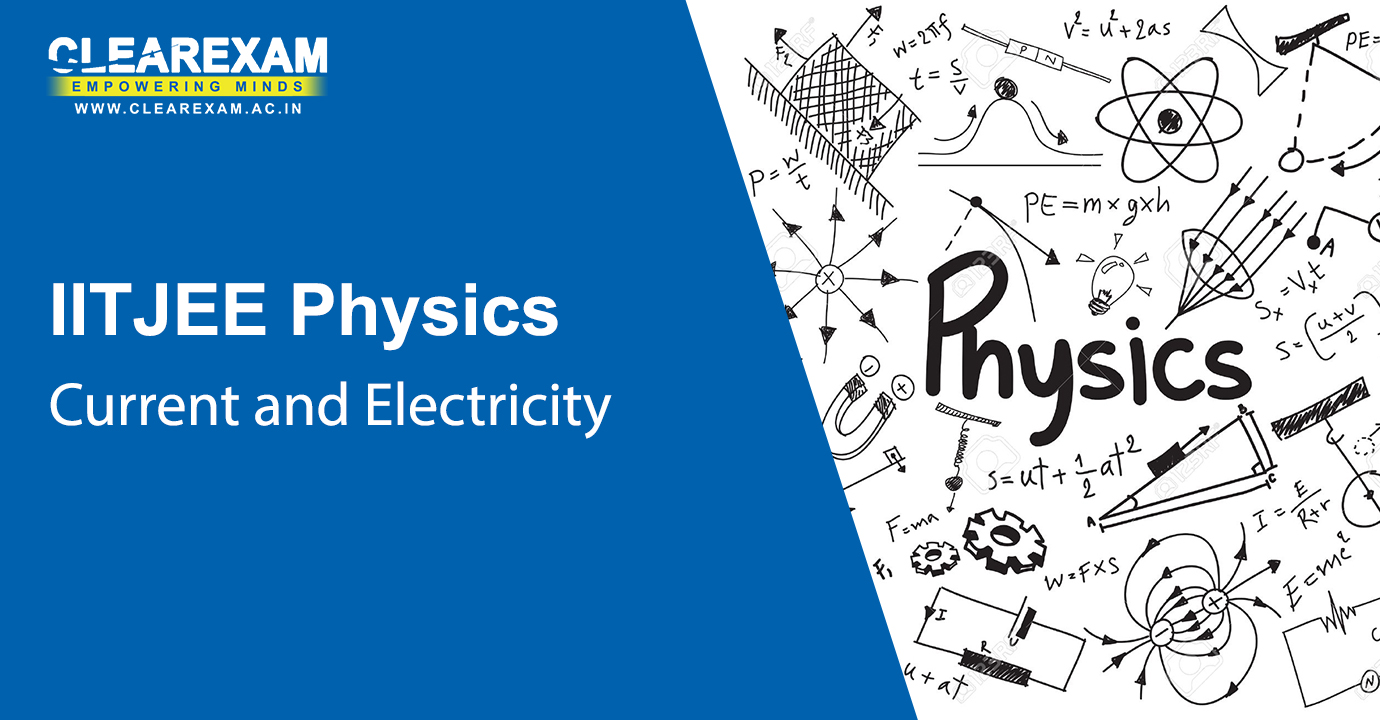Current Electricity
Learn from the experts through their NEET Physics Current Electricity Video Lectures consisting of thetopics Electric Current, Electric Currents in Conductors, Ohm’s Law, Drift of Electrons & the Origin of Resistivity, Limitations of Ohm’s Law, Resistivity of Various Materials, Temperature Dependence of Resistivity, Electrical Energy, Power, Combination of Resistors liked Series and Parallel, Cells, emf, Internal Resistance, Cells in Series and in Parallel, Kirchhoff’s Laws, Wheatstone Bridge, Meter Bridge and Potentiometer.
Electric Current and Current Density
Chapter - Current Electricity
Subject - NEET Physics
Drift Velocity and Resistance
Chapter - Current Electricity
Subject - NEET Physics
Mobility and Temperature Dependence of Resistivity
Chapter - Current Electricity
Subject - NEET Physics
Electromotive Force and Ohm's Law
Chapter - Current Electricity
Subject - NEET Physics
Electrical Energy and Power
Chapter - Current Electricity
Subject - NEET Physics
Series and Parallel Combination of Resistances
Chapter - Current Electricity
Subject - NEET Physics
Equivalent Circuits
Chapter - Current Electricity
Subject - NEET Physics
Series and Parallel Combinations of Cells
Chapter - Current Electricity
Subject - NEET Physics
Kirchhoff's Laws
Chapter - Current Electricity
Subject - NEET Physics
Wheatstone's Bridge, Meter Bridge and Potentiometer
Chapter - Current Electricity
Subject - NEET Physics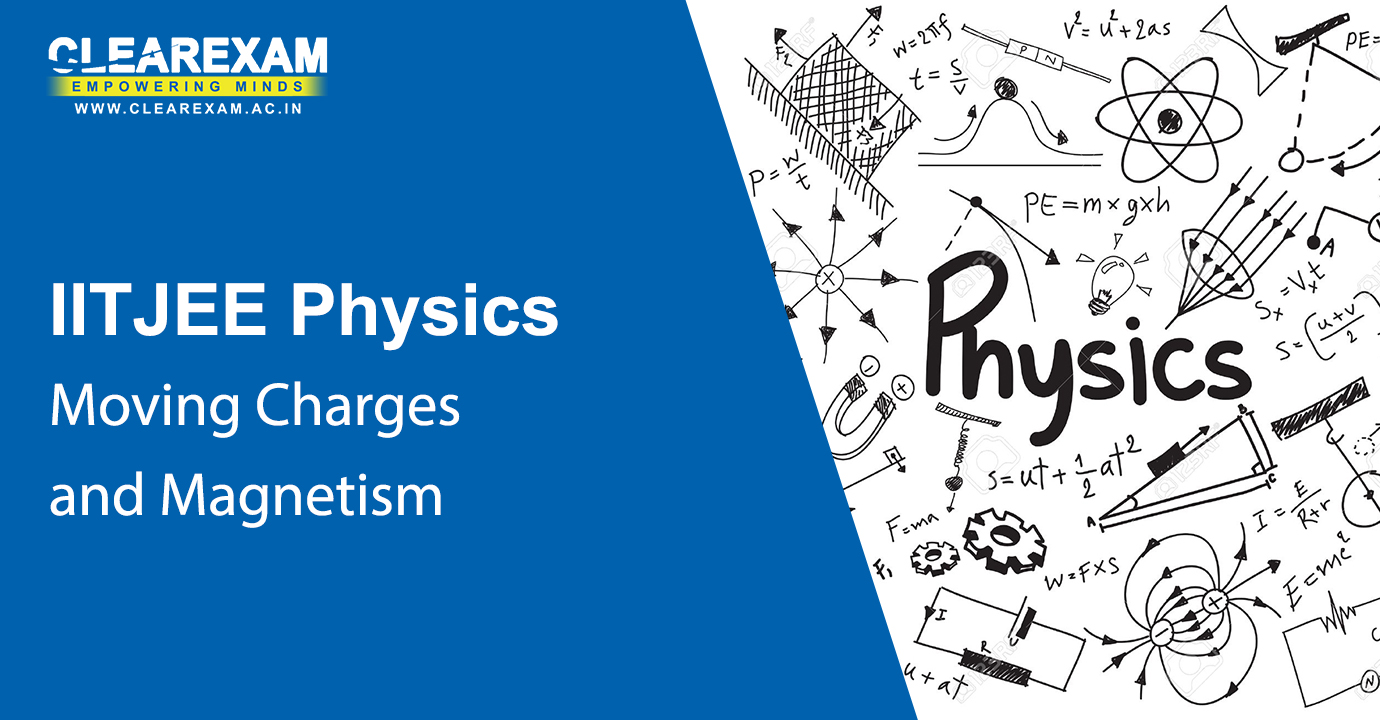Moving Charges and Magnetism
Wade through the syllabus with our NEET Physics Moving Charges and Magnetism Video Lectures  on the topics as given: Magnetic Force, Motion in a Magnetic Field, Motion in Combined Electric and Magnetic Fields, Magnetic Field due to a Current Element, Biot-Savart Law, Magnetic Field on the Axis of a Circular Current Loop, Ampere’s Circuital Law, the Solenoid & the Toroid, Force between Two Parallel Currents, the Ampere, Torque on a Current Loop, Magnetic Dipole and The Moving Coil Galvanometer.
Introduction to Magnetostatics, Biot Savart Law
Chapter - Moving Charges and Magnetism
Subject - NEET Physics
Motion of Charges in the Presence of Electric and Magnetic Fields
Chapter - Moving Charges and Magnetism
Subject - NEET Physics
Magnetic Field for a Straight Conductor and Ampere's Law
Chapter - Moving Charges and Magnetism
Subject - NEET Physics
Generalization of Ampere's Law and its Applications
Chapter - Moving Charges and Magnetism
Subject - NEET Physics
More Applications of Ampere's Law
Chapter - Moving Charges and Magnetism
Subject - NEET Physics
Force and Torque due to Magnetic Field
Chapter - Moving Charges and Magnetism
Subject - NEET Physics
Moving Coil Galvanometer, Magnetic Dipole
Chapter - Moving Charges and Magnetism
Subject - NEET Physics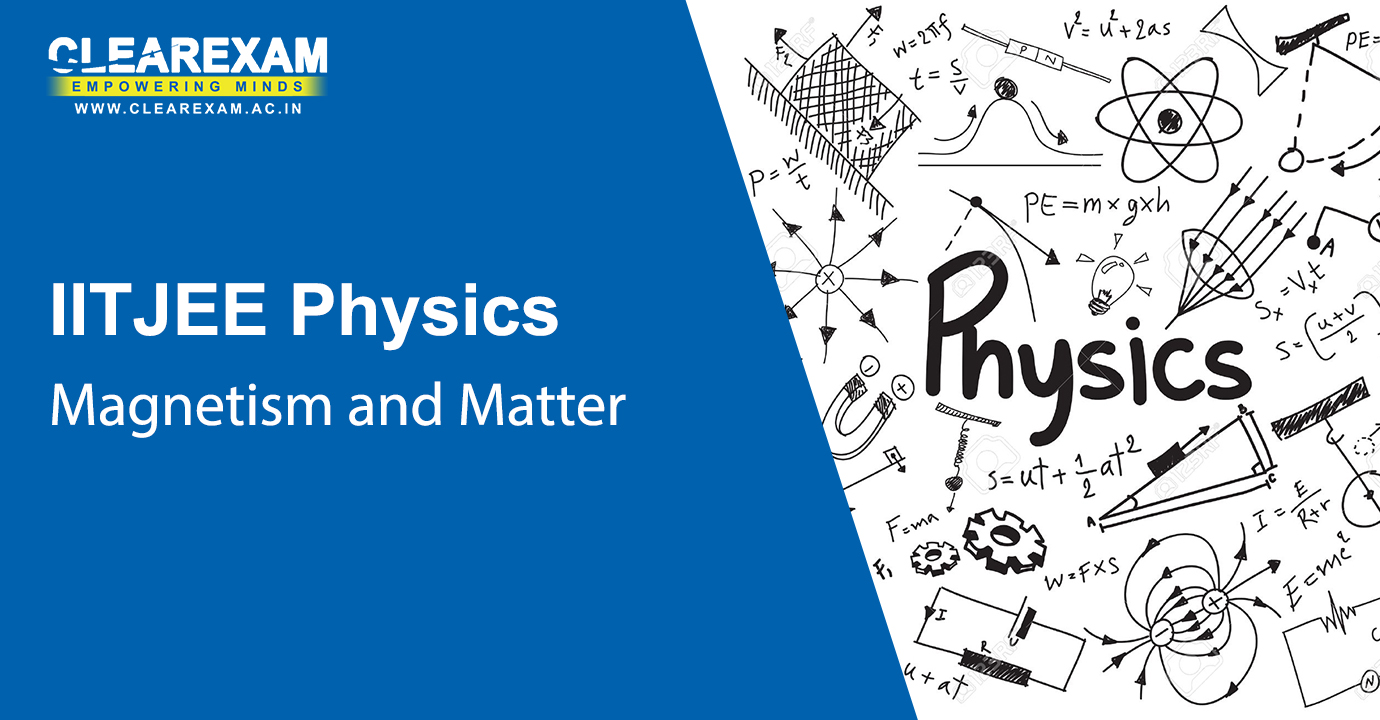Magnetism and Matter
Study smart with the NEET Physics Magnetism and Matter Video Lectures consisting of topics such as The Bar Magnet, Magnetism & Gauss’s Law, The Earth’s Magnetism, Magnetisation & Magnetic Intensity, Magnetic Properties of Materials and Permanent Magnets & Electromagnets.
Magnetic Dipole, Magnetization
Chapter - Magnetism and Matter
Subject - NEET Physics
Magnetization and Application of Ampere's Law
Chapter - Magnetism and Matter
Subject - NEET Physics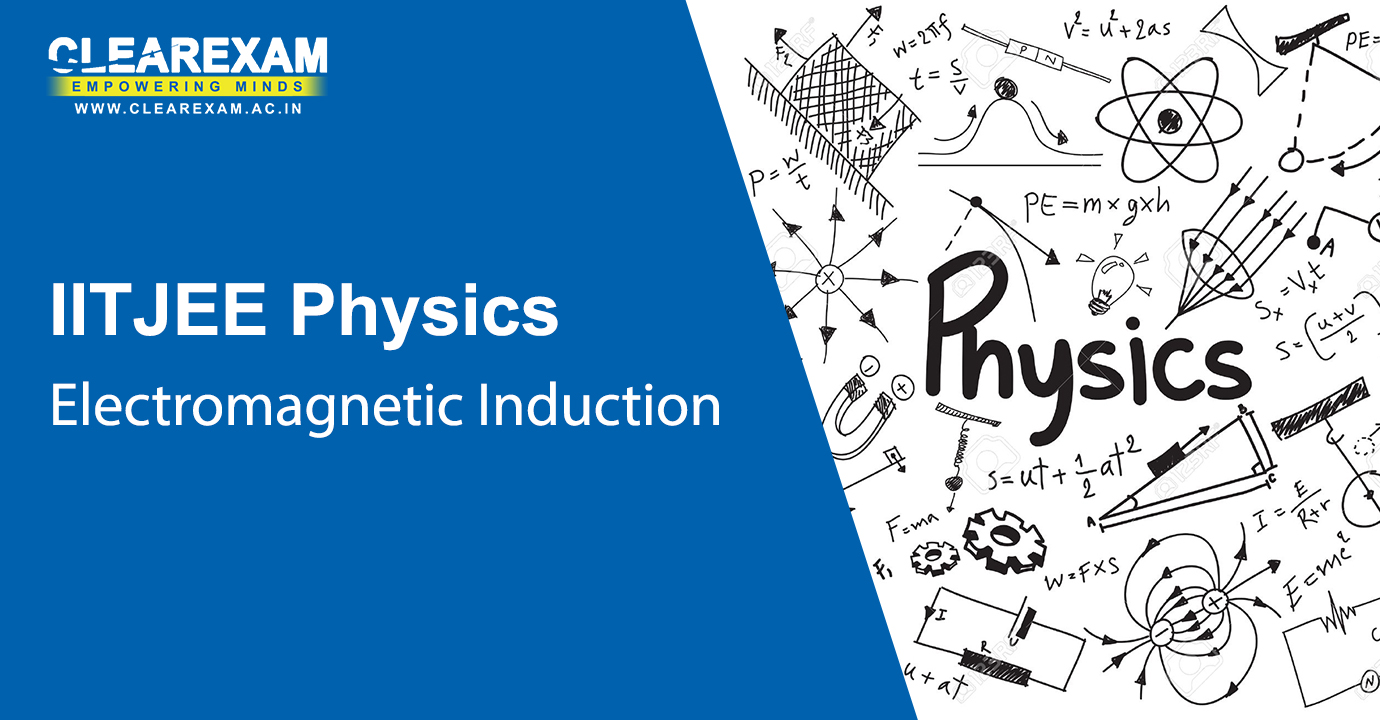Electromagnetic Induction
Get ready for an effective study by our NEET Physics Electromagnetic Induction Video Lectures, where the following topics have been explained : The Experiments of Faraday & Henry, Magnetic Flux, Faraday’s Law of Induction, Lenz’s Law & Conservation of Energy, Motional Electromotive Force, Energy Consideration A Quantitative Study, Eddy Currents, Inductance and AC Generator.
Electromagnetic Induction
Chapter - Electromagnetic Induction
Subject - NEET Physics
Faraday's Law of Induction, Induced EMF, Lenz's Law, Motional EMF
Chapter - Electromagnetic Induction
Subject - NEET Physics
Eddy Currents, Inductance, Mutual and Self-Inductance
Chapter - Electromagnetic Induction
Subject - NEET Physics
Energy in Magnetic Field
Chapter - Electromagnetic Induction
Subject - NEET Physics
AC Generator
Chapter - Electromagnetic Induction
Subject - NEET Physics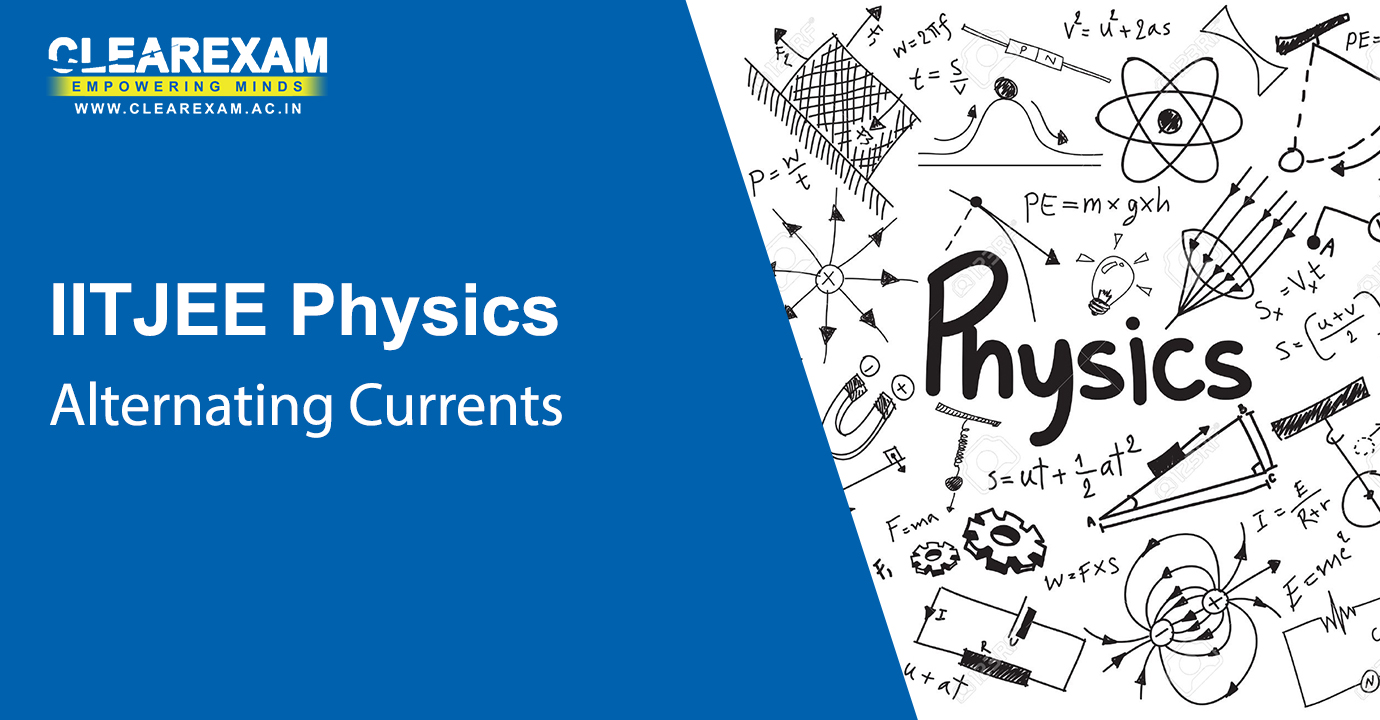Alternating Current
Utilize your 1 hour effectively with the NEET Physics Alternating Current Video Lectures comprising of the various topics such as AC Voltage Applied to a Resistor, Representation of AC Current & Voltage by Rotating Vectors -Phasors, AC Voltage Applied to an Inductor, AC Voltage Applied to a Capacitor, AC Voltage Applied to a Series LCR Circuit, Power in AC Circuit: The Power Factor, LC Oscillations and Transformers.
Circuits with Resistance and Inductance
Chapter - Alternating Current
Subject - NEET Physics
Capacitive Circuits
Chapter - Alternating Current
Subject - NEET Physics
LCR Circuit: Graphical Solution
Chapter - Alternating Current
Subject - NEET Physics
LCR Circuit: Analytical Solution, Resonance
Chapter - Alternating Current
Subject - NEET Physics
LCR Circuit: Applications
Chapter - Alternating Current
Subject - NEET Physics
LCR Circuit: Power Factor
Chapter - Alternating Current
Subject - NEET Physics
LC Oscillations
Chapter - Alternating Current
Subject - NEET Physics
Transformers
Chapter - Alternating Current
Subject - NEET PhysicsElectromagnetic Waves
In this part of our video lectures, the given NEET Physics Electromagnetic Waves Video Lectures consists of Displacement Current, Electromagnetic Waves and the Electromagnetic Spectrum. The topics have been explained in great detail by the top subject experts of the country.
Concepts of Waves and Electromagnetic Waves
Chapter - Electromagnetic Waves
Subject - NEET Physics
Maxwell's Equations and Electromagnetic Waves
Chapter - Electromagnetic Waves
Subject - NEET Physics
Displacement Current
Chapter - Electromagnetic Waves
Subject - NEET Physics
Problems in Electromagnetics: Electrostatics
Chapter - Electromagnetic Waves
Subject - NEET Physics
Problems in Electromagnetics: Magnetic Fields, EM Waves
Chapter - Electromagnetic Waves
Subject - NEET Physics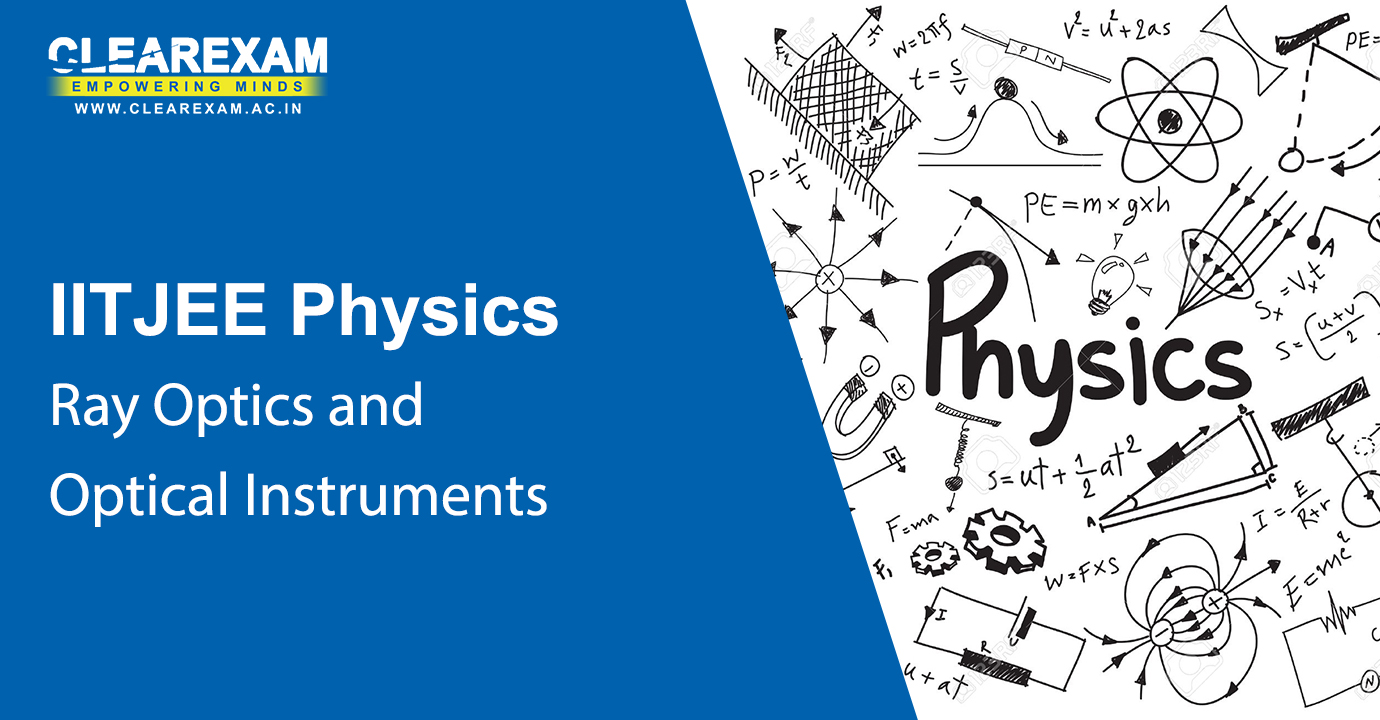Ray Optics and Optical Instruments
With NEET Physics Ray Optics and Optical Instruments Video Lectures ,consisting of the topics:Reflection of light by spherical mirrors, refraction, total internal reflection, refraction at spherical surfaces and by lenses, refraction through a prism, dispersion by a prism, some natural phenomena due to sun light and optical instruments, you will begin to see physics in a different light.
What is Light-1
Chapter - Ray Optics and Optical Instruments
Subject - NEET Physics
What is Light-2
Chapter - Ray Optics and Optical Instruments
Subject - NEET Physics
General Introduction
Chapter - Ray Optics and Optical Instruments
Subject - NEET Physics
Reflection of Light and Formation of Images
Chapter - Ray Optics and Optical Instruments
Subject - NEET Physics
The Mirror Equation
Chapter - Ray Optics and Optical Instruments
Subject - NEET Physics
Refraction of Light
Chapter - Ray Optics and Optical Instruments
Subject - NEET Physics
Total Internal Reflection
Chapter - Ray Optics and Optical Instruments
Subject - NEET Physics
Refraction at Spherical Surfaces and by Lenses
Chapter - Ray Optics and Optical Instruments
Subject - NEET Physics
Power of a Lens and Combination of Thin Lenses in Contact
Chapter - Ray Optics and Optical Instruments
Subject - NEET Physics
Refraction through a Prism and Dispersion
Chapter - Ray Optics and Optical Instruments
Subject - NEET Physics
Viewing Objects: Eye as an Optical Instrument
Chapter - Ray Optics and Optical Instruments
Subject - NEET Physics
Microscopes and Telescopes
Chapter - Ray Optics and Optical Instruments
Subject - NEET Physics
Resolving Power of Optical Instruments
Chapter - Ray Optics and Optical Instruments
Subject - NEET PhysicsWave Optics
Indulge in an effective prep , with our all-accessible video lectures. The NEET Physics Wave Optics Video Lectures has Huygens Principle, Refraction & Reflection of plane waves using Huygens Principle, Coherent & Incoherent Addition of Waves, interference of light waves and Young’s experiment, Diffraction, Polarisation, all well explained by the top IIT Professors.
Wave optics: Huygens Principle
Chapter - Wave Optics
Subject - NEET Physics
Young's Interference Experiment
Chapter - Wave Optics
Subject - NEET Physics
Interference with Coherent and Incoherent Waves
Chapter - Wave Optics
Subject - NEET Physics
Fringe Shift in the Two-hole Interference Equipment
Chapter - Wave Optics
Subject - NEET Physics
Diffraction
Chapter - Wave Optics
Subject - NEET Physics
Polarisation of Light
Chapter - Wave Optics
Subject - NEET PhysicsDual Nature of Matter and Radiation
Refer to the NEET Physics Dual Nature of Matter and Radiation Video Lectures , where the following topics have been discussed : electron emission, photoelectric effect, experimental study of photoelectric effect, photoelectric effect and wave theory of light, Einstein’s Photoelectric Equation: Energy Quantum of Radiation, particle nature of light: the photon, wave nature of matter, Davisson and Germer Experiment. Learn the topics in an engaging manner and retain them till the D-day.
Modern Physics: General Introduction
Chapter - Dual Nature of Matter and Radiation
Subject - NEET Physics
Modern Physics-1
Chapter - Dual Nature of Matter and Radiation
Subject - NEET Physics
Modern Physics-2
Chapter - Dual Nature of Matter and Radiation
Subject - NEET Physics
Photoelectric Effects: Facts and Prospects
Chapter - Dual Nature of Matter and Radiation
Subject - NEET Physics
Photoelectric Effect: Einstein's Explanation
Chapter - Dual Nature of Matter and Radiation
Subject - NEET Physics
Wave Nature of Matter
Chapter - Dual Nature of Matter and Radiation
Subject - NEET Physics
Matter Waves & Structure of the Atom
Chapter - Dual Nature of Matter and Radiation
Subject - NEET Physics
Rutherford Scattering and Introduction to Bohr Model
Chapter - Dual Nature of Matter and Radiation
Subject - NEET PhysicsAtoms
Want to know all about Atoms, then buck up with the NEET Physics Atoms Video Lectures. It comprises oftopics such as Alpha-particle Scattering and Rutherford’s Nuclear Model of Atom, Atomic Spectra, Bohr Model of the Hydrogen Atom, The Line Spectra of the Hydrogen Atom and De-Broglie’s Explanation of Bohr’s Second Postulate of Quantisation.
Structure of the Atom
Chapter - Atoms
Subject - NEET Physics
Atomic Models
Chapter - Atoms
Subject - NEET Physics
Bohr Model of Atom-1
Chapter - Atoms
Subject - NEET Physics
Bohr Model of Atom-2
Chapter - Atoms
Subject - NEET PhysicsNuclei
Enhance your understanding about the Nuclei, with the NEET Physics Nuclei Video Lectures with topics such as Atomic Masses and Composition of the Nucleus, Size of the Nucleus, Nuclear Force, Radioactivity and Nuclear Energy.
Atomic Nucleus-1
Chapter - Nuclei
Subject - NEET Physics
Atomic Nucleus-2
Chapter - Nuclei
Subject - NEET Physics
Atomic Nucleus: Masses & Stability-1
Chapter - Nuclei
Subject - NEET Physics
Atomic Nucleus: Masses & Stability-2
Chapter - Nuclei
Subject - NEET Physics
Chapter - Nuclei
Subject - NEET PhysicsSemiconductor Electronics
Interested in Semiconductors ? Then trot along with the NEET Physics Semiconductor Electronics Video Lectures. It covers the following topics : Classification of Metals, Conductors & Semiconductors, Intrinsic Semiconductor, Extrinsic Semiconductor, p-n Junction, semiconductor diode, application of junction diode as a rectifier, special purpose, p-n junction diodes, junction transistor, digital electronics & logic gates and integrated circuits.
Conductors, Semiconductors and Insulators
Chapter - Semiconductor Electronics
Subject - NEET Physics
Doping in Semiconductors
Chapter - Semiconductor Electronics
Subject - NEET Physics
P-N Junction Basics
Chapter - Semiconductor Electronics
Subject - NEET Physics
Field and Potential in P-N Junction
Chapter - Semiconductor Electronics
Subject - NEET Physics
Current through a P-N Junction
Chapter - Semiconductor Electronics
Subject - NEET Physics
Special Purpose P-N Junction
Chapter - Semiconductor Electronics
Subject - NEET Physics
Bipolar Junction Transistor Basics
Chapter - Semiconductor Electronics
Subject - NEET Physics
Transistor as an Amplifier and as a Switch
Chapter - Semiconductor Electronics
Subject - NEET PhysicsCommunication Systems
Engage in these 1 hour video sessions of the NEET Physics Communication Systems Video Lectures. It has topics like elements of a communication system, basic terminology used in electronic communications systems, bandwidth of signals, bandwidth of transmission medium, propagation of electromagnetic waves, modulation & its necessity, amplitude modulation, production of amplitude modulated wave and detection of amplitude modulated wave.
Basics of Electronic Communication Systems: Modulation and its necessity
Chapter - Communication Systems
Subject - NEET Physics
Detection of Amplitude Modulated Waves
Chapter - Communication Systems
Subject - NEET PhysicsExperimental Physics
In this section of the video series comprising of the NEET Physics Experimental Physics Video Lectures, the student gets a holistic understanding of all the experiments that he or she has performed during the lab sessions. Get a gist of the core concepts involved in the making of these experiments.
Simple Experiments Based on Basic Principles of Mechanics
Chapter - Experimental Physics
Subject - NEET Physics

## Want to know more

Want to Know More
Please fill in the details below:

Name